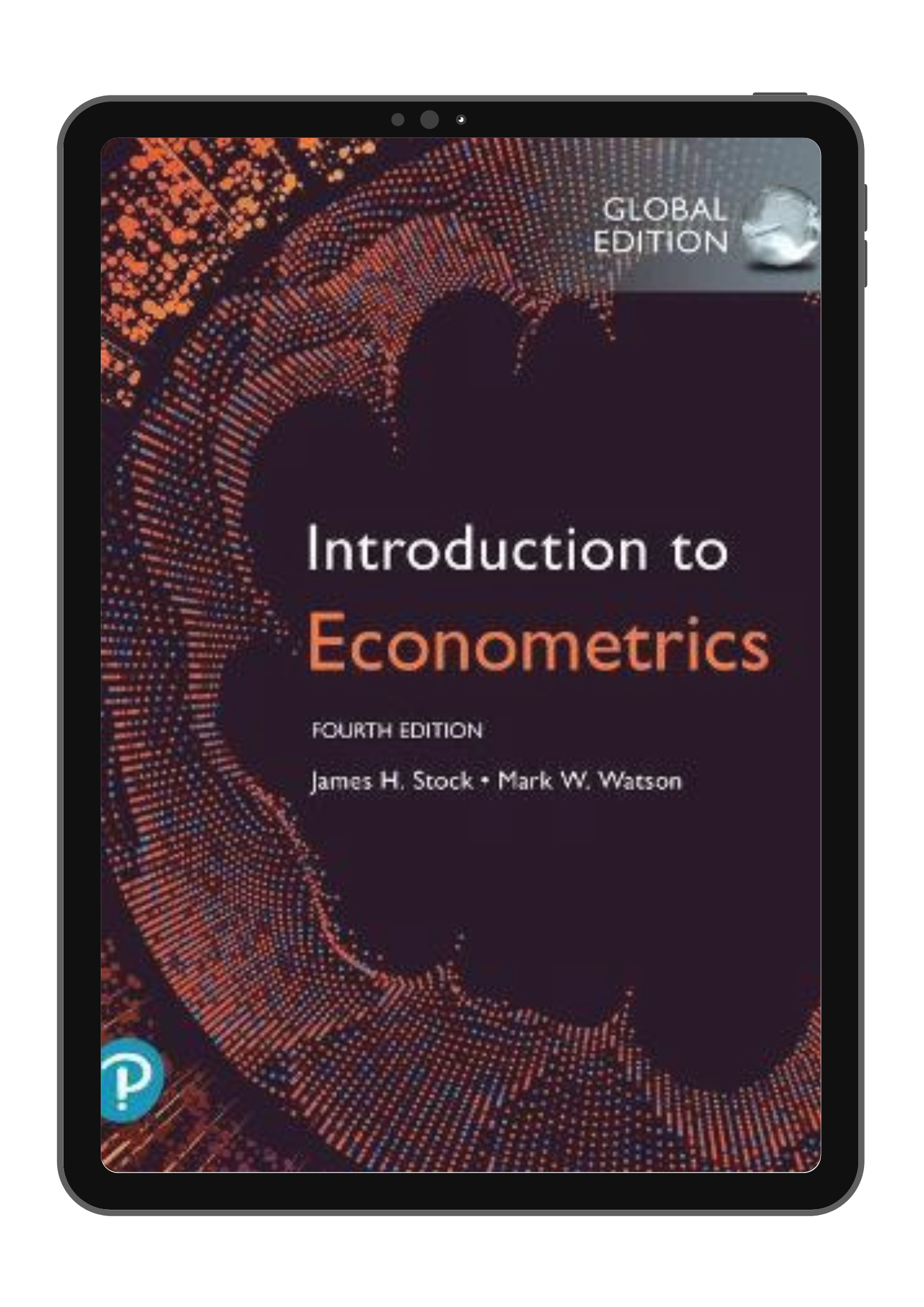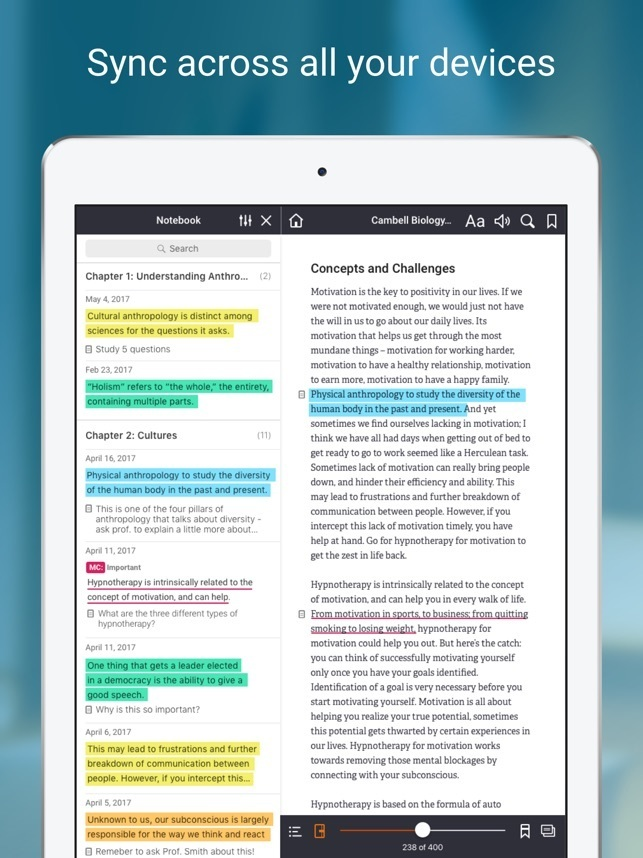••••# (EBook) Introduction to Econometrics 4E By James Stock 9781292264523

RM 76.00
- +

PART I: INTRODUCTION AND REVIEW
1. Economic Questions and Data
2. Review of Probability
3. Review of Statistics
PART II: FUNDAMENTALS OF REGRESSION ANALYSIS
4. Linear Regression with One Regressor
5. Regression with a Single Regressor: Hypothesis Tests and Confidence Intervals
6. Linear Regression with Multiple Regressors
7. Hypothesis Tests and Confidence Intervals in Multiple Regression
8. Nonlinear Regression Functions
9. Assessing Studies Based on Multiple Regression
PART III: FURTHER TOPICS IN REGRESSION ANALYSIS
10. Regression with Panel Data
11. Regression with a Binary Dependent Variable
12. Instrumental Variables Regression
13. Experiments and Quasi-Experiments
14. Prediction with Many Regressors and Big Data
PART IV: REGRESSION ANALYSIS OF ECONOMIC TIME SERIES DATA
15. Introduction to Time Series Regression and Forecasting
16. Estimation of Dynamic Causal Effects
17. Additional Topics in Time Series Regression
PART V: THE ECONOMIC THEORY OF REGRESSION ANALYSIS
18. The Theory of Linear Regression with One Regressor
19. The Theory of Multiple Regression

Delivery Policy

This is when the ordering is in the form of :

For E-Books or Online Leaning Access Code

Format : Access Code  ( Digital  )

The code will be sending to your email in 2 to 3 working days normally .

It is very important that you include you email and your contact number correctly.

All Product have a expiry date of 12 month . The time will only start once the code is activated.

The instruction on how to download will be send to you with the code together.

**All access code and EBook code once order CAN'T be return.**